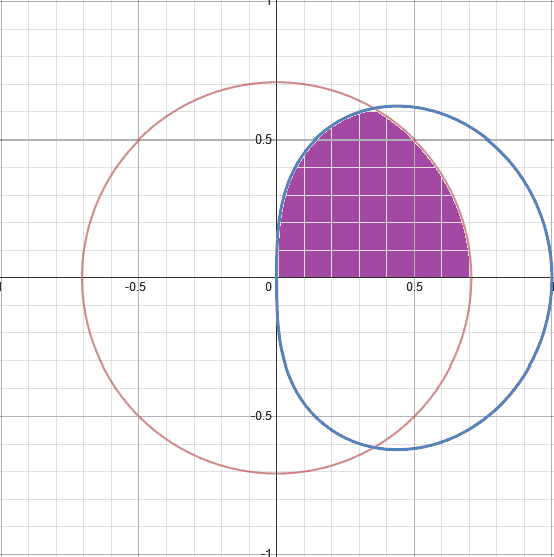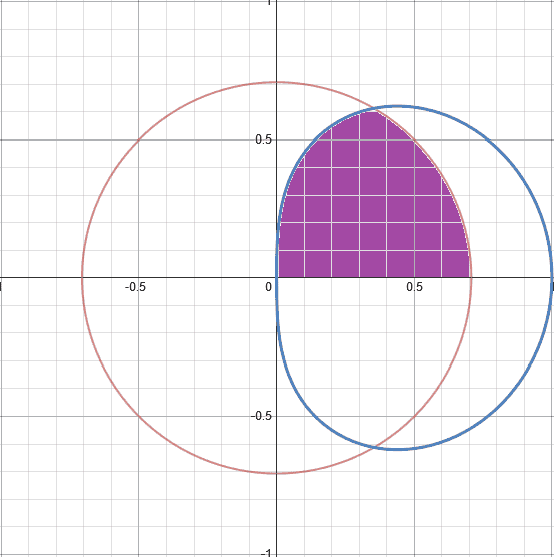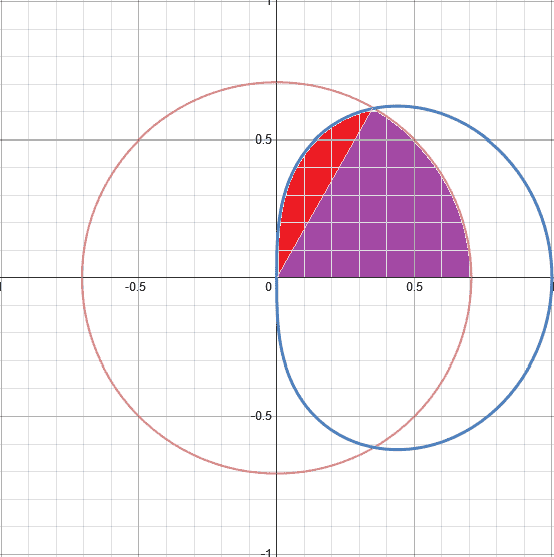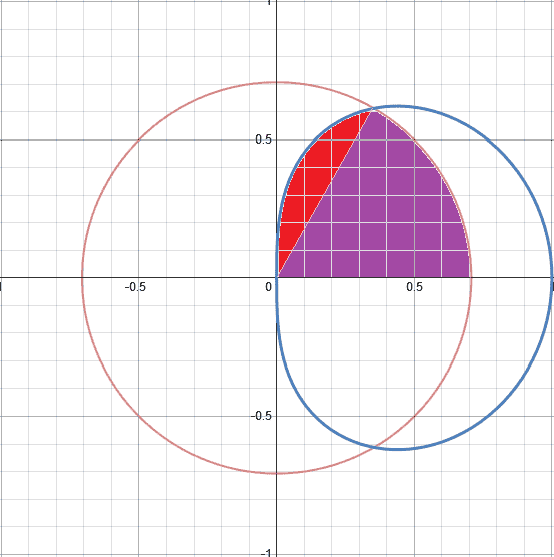# How to find the area enclosed by two polar curves?

Eclair_de_XII

## Homework Statement

"Areas of regions Make a sketch of the region and its bounding curves. Find the area of the region."
"The region inside the curve ##r = \sqrt{cosθ}## and inside the circle ##r = \frac{\sqrt{2}}{2}##.

## Homework Equations

##A = \frac{1}{2}\int_α^β(f(θ)^2-g(θ)^2)dθ##
Answer as given by textbook: ##\frac{1}{4}(2-\sqrt{3})+\frac{π}{12}##

## The Attempt at a SolutionNow, since the problem is asking for the region shared by both curves in the first quadrant, the lower limit of my integral would be 0, up until some arbitrary point which I solved for by equating the two curves.

##\sqrt{cosθ} = \frac{\sqrt{2}}{2}##
##cosθ=\frac{1}{2}##
##θ=arccos(\frac{1}{2})=\frac{π}{3}##

So my upper limit would be ##\frac{π}{3}##. Plugging these limits into the equation, I have...

##\frac{1}{2}\int_0^{\frac{π}{3}}(\frac{1}{2}-cosθ)dθ = (\frac{1}{4}θ-\frac{1}{2}sinθ)|_0^{\frac{π}{3}} = \frac{π}{12}-\frac{\sqrt{3}}{4} = \frac{π}{12}-\frac{1}{4}(\sqrt{3})##

So I'm missing like a ##\frac{1}{2}## in my answer, and I don't know why. Could someone tell me what I did wrong?

Homework Helper
Gold Member

## Homework Equations

##A = \frac{1}{2}\int_α^β(f(θ)^2-g(θ)^2)dθ##
Why are you using that equation? That would be the equation for the area between the two curves. But the purple area in your diagram is, for every radial line, only bounded by one curve.

Staff Emeritus
Homework Helper

## Homework Statement

"Areas of regions Make a sketch of the region and its bounding curves. Find the area of the region."
"The region inside the curve ##r = \sqrt{cosθ}## and inside the circle ##r = \frac{\sqrt{2}}{2}##.

## Homework Equations

##A = \frac{1}{2}\int_α^β(f(θ)^2-g(θ)^2)dθ##
Answer as given by textbook: ##\frac{1}{4}(2-\sqrt{3})+\frac{π}{12}##

## The Attempt at a SolutionNow, since the problem is asking for the region shared by both curves in the first quadrant, the lower limit of my integral would be 0, up until some arbitrary point which I solved for by equating the two curves.

##\sqrt{cosθ} = \frac{\sqrt{2}}{2}##
##cosθ=\frac{1}{2}##
##θ=arccos(\frac{1}{2})=\frac{π}{3}##

So my upper limit would be ##\frac{π}{3}##. Plugging these limits into the equation, I have...

##\frac{1}{2}\int_0^{\frac{π}{3}}(\frac{1}{2}-cosθ)dθ = (\frac{1}{4}θ-\frac{1}{2}sinθ)|_0^{\frac{π}{3}} = \frac{π}{12}-\frac{\sqrt{3}}{4} = \frac{π}{12}-\frac{1}{4}(\sqrt{3})##

So I'm missing like a ##\frac{1}{2}## in my answer, and I don't know why. Could someone tell me what I did wrong?
Look carefully at the figure you drew.

The angle π/3 gets you to the intersection of these two curves, but have you accounted for all of the area inside the overlap of these two curves?

Eclair_de_XII
That would be the equation for the area between the two curves. But the purple area in your diagram is, for every radial line, only bounded by one curve.

It looks to me that it is bounded by the y-axis and either ##r = \frac{\sqrt{2}}{2}## or ##r = cosθ##. So it can only be bounded by one of these, depending on which function I chose for my inner and outer curves? In this case, ##r = \frac{\sqrt{2}}{2}## is my outer and ##r = cosθ## is my inner curve?

The angle π/3 gets you to the intersection of these two curves, but have you accounted for all of the area inside the overlap of these two curves?

You're right; the area is bounded by ##(0,\frac{π}{2})##. Right now, I think I only solved for the purple section of the overlapping curves, as shown below.So I would need to solve for that red portion, which is supposed to be ##\frac{1}{2}##. I'm thinking of keeping my integral as it is, and just adding the following integral to it: ##\frac{1}{2}\int_{\frac{π}{3}}^{\frac{π}{2}}(cos^2θ-g(θ))dθ##, where ##g(θ)## is the slope from the origin to the point of intersection. I'm going to get to work on figuring out how to express lines using polar coordinates, unless someone has a say in the efficiency of this method. Additionally, if anyone knows how to shrink images, that would also be appreciated.

Homework Helper
Gold Member
It looks to me that it is bounded by the y-axis and either ##r = \frac{\sqrt{2}}{2}## or ##r = cosθ##. So it can only be bounded by one of these, depending on which function I chose for my inner and outer curves? In this case, ##r = \frac{\sqrt{2}}{2}## is my outer and ##r = cosθ## is my inner curve?
No. There is no outer and inner curve, only an outer one. The outer curve is the circle for ##\theta## from 0 to the intersection point you identified - that gives you the purple area. The outer curve is the ##(\sqrt{\cos\theta},\theta)## curve for ##\theta## from there to ##\pi/2##. That gives you the red area.
I'm thinking of keeping my integral as it is, and just adding the following integral to it: ##\frac{1}{2}\int_{\frac{π}{3}}^{\frac{π}{2}}(cos^2θ-g(θ))dθ##, where ##g(θ)## is the slope from the origin to the point of intersection.
No, don't keep any integral that was done in the form ##\int_a^b (f(\theta)^2-g(\theta)^2)d\theta##. They are based on the area between an outer and inner curve and there is no inner curve. Get rid of the ##g(\theta)^2## part.

•Eclair_de_XII
Staff Emeritus
Homework Helper
It looks to me that it is bounded by the y-axis and either ##r = \frac{\sqrt{2}}{2}## or ##r = cosθ##. So it can only be bounded by one of these, depending on which function I chose for my inner and outer curves? In this case, ##r = \frac{\sqrt{2}}{2}## is my outer and ##r = cosθ## is my inner curve?

You're right; the area is bounded by ##(0,\frac{π}{2})##. Right now, I think I only solved for the purple section of the overlapping curves, as shown below.So I would need to solve for that red portion, which is supposed to be ##\frac{1}{2}##. I'm thinking of keeping my integral as it is, and just adding the following integral to it: ##\frac{1}{2}\int_{\frac{π}{3}}^{\frac{π}{2}}(cos^2θ-g(θ))dθ##, where ##g(θ)## is the slope from the origin to the point of intersection. I'm going to get to work on figuring out how to express lines using polar coordinates, unless someone has a say in the efficiency of this method. Additionally, if anyone knows how to shrink images, that would also be appreciated.
You don't need to figure out the equation of the line separating the red area from the purple area.

In polar coordinates, a point is defined by two pieces of data: r and θ.

The red area is the area of the curve
$$r = \sqrt{cos (θ)}$$ between θ = π/3 and θ = π/2.•Eclair_de_XII
Eclair_de_XII
No. There is no outer and inner curve, only an outer one. The outer curve is the circle for ##\theta## from 0 to the intersection point you identified - that gives you the purple area. The outer curve is the ##(\sqrt{\cos\theta},\theta)## curve for ##\theta## from there to ##\pi/2##. That gives you the red area.
No, don't keep any integral that was done in the form ##\int_a^b (f(\theta)^2-g(\theta)^2)d\theta##

So what form of integral should I use in order to find that red area, if I am unable to use the one you listed as bad?

Homework Helper
Gold Member
Do you not have a formula for the area with a single curve? It would be very odd for your teachers to give you a formula for the case where there are two curves and not for where there is one.

Also, rough estimation shows the following cannot be even roughly correct:
So I would need to solve for that red portion, which is supposed to be ##\frac12##
Each little square in the diagram has area 0.01. Approx how many little squares fit inside the red area? Far fewer than 50.

Estimation can be helpful in cases like this, to check the reasonableness of answers and that you are staying on the right track.

Eclair_de_XII
The red area is the area of the curve

##r = \sqrt{cos (θ)}##

between ##θ = π/3## and ##θ = π/2##.Hm, I think I get what you're saying. ##\frac{1}{2}\int_\frac{π}{3}^\frac{π}{2}(cos^\frac{1}{2}(θ))^2dθ=\frac{1}{2}\int_\frac{π}{3}^\frac{π}{2}(cos(θ))dθ = \frac{1}{2}sin(θ)|_\frac{π}{3}^\frac{π}{2}=\frac{1}{2}(sin(\frac{π}{2})-sin(\frac{π}{3}))=\frac{1}{2}[1-\frac{\sqrt{3}}{2})]=\frac{1}{2}(1-\frac{\sqrt{3}}{2})=\frac{1}{4}(2-\sqrt{3})##. So now, that's one part of the equation, and now that's left is the ##\frac{\pi}{12}## part, which I already solved for in my original post, alongside the ##-\frac{\sqrt{3}}{4}##. Now, to solve for the ##\frac{\pi}{12}##, I think I should just integrate the ##r = \frac{\sqrt{2}}{2}##, as such: ##\frac{1}{2}\int_0^{\frac{\pi}{3}}\frac{1}{2}dθ=\frac{1}{4}|_0^\frac{\pi}{3}=\frac{\pi}{12}##.

Do you not have a formula for the area with a single curve?

I'm self-studying for the Calculus III course I'm going to be taking in a month, so I'm just teaching myself from the book in the meantime. Anyway, it did not give a formula to solve for the area of a single curve; just for areas between two distinct curves. Though, if I had to guess what that formula was, I would think to set the ##g(x)## of the equation I posted to zero, and let the integral become: ##\frac{1}{2}\int_α^β(f(x))^2dx##. I'm probably mistaken, though. Anyway, thanks for the help, Steam and andrew. You've been a big help; I appreciate it.

Staff Emeritus
Homework Helper
I'm self-studying for the Calculus III course I'm going to be taking in a month, so I'm just teaching myself from the book in the meantime. Anyway, it did not give a formula to solve for the area of a single curve; just for areas between two distinct curves. Though, if I had to guess what that formula was, I would think to set the ##g(x)## of the equation I posted to zero, and let the integral become: ##\frac{1}{2}\int_α^β(f(x))^2dx##. I'm probably mistaken, though. Anyway, thanks for the help, Steam and andrew. You've been a big help; I appreciate it.

You can always supplement the text you are studying with additional materials if some of the concepts are not presented too clearly.

Paul's Online Math Notes give pretty concise instruction in calculus, differential equations and some of the basic math leading up to these.

Here is Paul's section on finding areas using polar coordinates:

http://tutorial.math.lamar.edu/Classes/CalcII/PolarArea.aspx

Eclair_de_XII